Engineering Mechanics - Kinematics of Particle (KOP)

Why Engineering Mechanics Kinematics of Particle (KOP)?

In this section you can learn and practice Engineering Mechanics Questions based on "Kinematics of Particle (KOP)" and improve your skills in order to face the interview, competitive examination and various entrance test (CAT, GATE, GRE, MAT, Bank Exam, Railway Exam etc.) with full confidence.

Where can I get Engineering Mechanics Kinematics of Particle (KOP) questions and answers with explanation?

IndiaBIX provides you lots of fully solved Engineering Mechanics (Kinematics of Particle (KOP)) questions and answers with Explanation. Solved examples with detailed answer description, explanation are given and it would be easy to understand. All students, freshers can download Engineering Mechanics Kinematics of Particle (KOP) quiz questions with answers as PDF files and eBooks.

Where can I get Engineering Mechanics Kinematics of Particle (KOP) Interview Questions and Answers (objective type, multiple choice)?

Here you can find objective type Engineering Mechanics Kinematics of Particle (KOP) questions and answers for interview and entrance examination. Multiple choice and true or false type questions are also provided.

How to solve Engineering Mechanics Kinematics of Particle (KOP) problems?

You can easily solve all kind of Engineering Mechanics questions based on Kinematics of Particle (KOP) by practicing the objective type exercises given below, also get shortcut methods to solve Engineering Mechanics Kinematics of Particle (KOP) problems.

Exercise :: Kinematics of Particle (KOP) - General Questions

• Kinematics of Particle (KOP) - General Questions
1.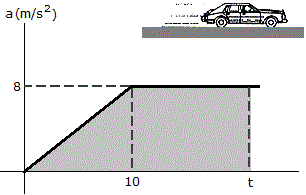A race car starting from rest moves along a straight track with an acceleration as shown in the graph (where for t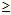10 s, a = 8 m/s2). Determine the time t for the car to reach a speed of 50 m/s.

 A. t = 11.25 s B. t = 6.25 s C. t = 12.5 s D. t = 3.53 s

Explanation:

No answer description available for this question. Let us discuss.

2.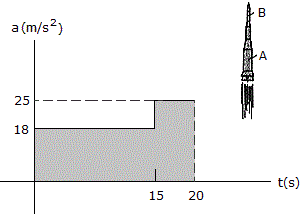A two-stage missile is fired vertically from rest with an acceleration as shown in the graph. In 15 s the first stage A burns out and the second stage B ignites. How fast is the rocket moving and how far has it gone at t = 20 s? How fast is the missile moving and how far has it gone at t = 20 s?

 A. v = 430 m/s, s = 4.30 km B. v = 395 m/s, s = 3.69 km C. v = 360 m/s, s = 3.60 km D. v = 500 m/s, s = 5.00 km

Explanation:

No answer description available for this question. Let us discuss.

3.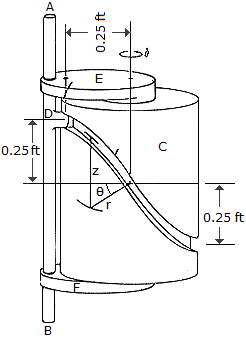The cylindrical cam C is held fixed while the rod AB and bearings E and F rotate about the vertical axis of the cam at a constant rate of= 4 rad/s. If the rod is free to slide through the bearings, determine the magnitudes of the velocity and acceleration of the guide D on the rod as a function of. The guide follows the groove in the cam, and the groove is defined by the equations r = 0.25 ft and z = (0.25 cos) ft.

 A.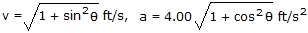B. v = cosft/s, a = 4.00 sinft/s2 C. v = sinft/s, a = 4.00 cosft/s2 D. v = 1.000 ft/s, a = 4.00 ft/s2

Explanation:

No answer description available for this question. Let us discuss.

4.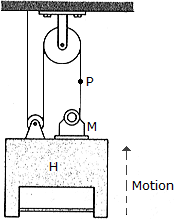If the hoist H is moving upward at 6 ft/s, determine the speed at which the motor M must draw in the supporting cable.

 A. VP/H = 6 ft/s B. VP/H = 2 ft/s C. VP/H = 12 ft/s D. VP/H = 18 ft/s

Explanation:

No answer description available for this question. Let us discuss.

5.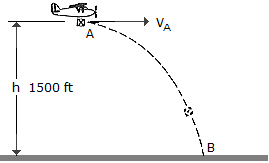A package is dropped from the plane which is flying with a constant horizontal velocity of vA = 150 ft/s at a height h = 1500 ft. Determine the radius of curvature of the path of the package just after it is released from plane at A.

 A.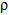2 = 9860 ft B.2 = 3000 ft C.2 = 1500 ft D.2 = 8510 ft